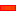﻿ Kinematics: Geared-Rocker

# Geared-Rocker

### Geared-Rocker Part

A Geared-Rocker Part is a kinematically-defined Part.

MechDesigner derives the motion for the Geared-Rocker Part from the:

 1 Motion of the DriveR-Part, relative to the Line-of-Centres between the two Gears

The Input Gear is a kinematically-defined Part and also a

 2 The number-of-teeth on each Gear
 3 Mesh of the Gear-Pair: Internal or External Mesh.

You must define the number-of-gear teeth and mesh in the Gear-Pair dialog-box.

####Geared-Rocker: Degrees-of-Freedom and Mobility

 Gruebler Equation: F = 3*(N-1) – 2*L- H F = 3 * (2-1) – 2*1 – 1 F = 3 – 2  –1 F= 0 Degrees-of-Freedom = 0 Parts N = 2. There are two parts: the Part we wish to specify as a Geared-Rocker and the kinematically-defined Part to which it is joined. Lower Pairs (Joints) L = 1 There is one Pin-Joint. Higher Pairs (Cams or Gears) H = 1 There is one Gear-Pair Therefore, a Gear-Pair and Geared-Rocker does not change the degrees-of-freedom of a kinematic-chain. It also means you can add as many Gear-Pairs as you want to a kinematic-chain. The number of Degrees-of-Freedom does not change.

####The Geared-Rocker in the Kinematics TreeA Geared-Rocker is constructed with a:• Part – see Add Part• Pin-Joint – see Add Pin-Joint• Gear-Pair – see Add Gear-Pair

####Preparation of elements before you can add a Gear-Pair

Simple Gear-Pair PreparationThe Part does not need to be a Rocker.

There must be three elements: Two kinematically-defined Parts, with a Linesketch-element in each, joined with a Pin-Joint.

A Rocker joined to a Line in the Base-Part is an example.

Step 1 is complete and the condition is satisfied.STEP 2: If necessary, start the Part-Editor to edit the Line and add a dimensionto the length of the Line

Step 2 is complete

 STEP 3: Close the Part-EditorSTEP 4: Add a PartSTEP 5: Join it with a Pin-Jointto the other end of the Line.

Step 5 is complete

It has a Blue Part-Outline.

Now you can use Add Gear-Pair. The Blue Part becomes the Geared-Rocker.

Epicyclic Gear-Pair PreparationSTEP 1: Join two Points with a Pin-Joint, with the Points at the ends of two Lines in kinematically-defined PartsEach Line sketch-element must be a child to the Parts that are kinematically-defined.

Simple example: a Rocker joined to a Line in the Base-Part.

Step 1 is complete and the condition is satisfied.STEP 2: Edit the Line to add a dimensionto the length of the Line

The CAD-Line in the Rocker has a dimension. Therefore, you do not need to add or edit the dimension.

 STEP 3: Close the Part-EditorSTEP 4: Add a PartSTEP 5: Join it with a Pin-Jointto the other end of the Line.

Step 5 is complete

It has a Blue Part-Outline.

Now you can use Add Gear-Pair. The Blue Part becomes the Geared-Rocker.

Tutorial and Reference Help Files for MechDesigner and MotionDesigner 13.2 + © Machine, Mechanism, Motion and Cam Design Software by PSMotion Ltd